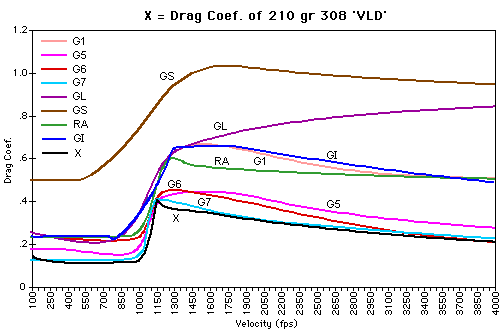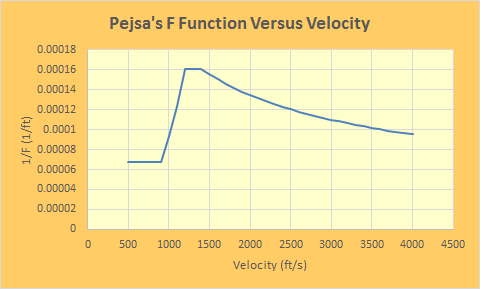# Pejsa's Projectile Drop Versus Distance Formula (Part 3 of 3)

The Roman emperor creates catastrophes for Rome and calls them successes.

— Celtic warrior's words about the Romans. Sounds like today's politicians.

## Introduction

In this post (part 3), I will work an example from Pejsa's "Modern Practical Ballistics 2nd ed." and show that the exact and approximate solutions to the drop differential equation give nearly the same answers.

While the results presented here are accurate for a projectile fired horizontally, projectiles are normally aimed using sights that are "zeroed" for specific ranges (Figure 2). Most drop versus range tables assume the rifle is zeroed at a specific range.

Zeroing involves adjusting your sight so that the projectile' s trajectory is given a slight upward tilt, which means the rifle's aim can be adjusted so that the bullet will strike where it is aimed for a specific range value.

Pejsa presents a modification of his horizontal drop formula that accounts for the effect of zeroing, but I will not be covering that material here – the extension is straightforward but a bit tedious. It may be material for a later post.

## Background

### Reference Projectiles

Pejsa's ballistic formulas are closely tied to empirical ballistic work done by Mayevski and Ingalls back in the 1800s (reference). The key idea behind the use of reference projectiles is that the effect of drag on a projectiles of the same shape but different masses and sizes can be related through a number called the ballistic coefficient.

The reference testing was performed using projectiles with 1 inch diameter and l pound mass (7000 grains). While numerous projectile shapes have been tested (Figure 5a shows drag data for some), only two shapes are commonly used for rifle ballistics – the G1 and G7 (Figure 3).

Pejsa argues the G7 data is the most applicable to modern spitzer bullet shapes (e.g. Figure 4) and his empirical formulas are based on the G7 data from Ingalls, slightly modified to remove some discontinuities present in the original work.

Appendix A contains a discussion of the reference data that Pejsa used to develop his ballistic formulas. Appendix B contains the actual table that he presented in his book.

### Ballistic Coefficient

The reference projectiles are enormous in mass and diameter relative to the bullets used by the shooting public. We will scale the reference projectile data using a number called the ballistic coefficient, which is defined here.

Ballistic Coefficient (BC)
In ballistics, the BC of a body is a measure of its ability to overcome air resistance in flight. It is inversely proportional to the negative acceleration — a high number indicates a low negative acceleration. BC is a function of mass, diameter, and drag coefficient. By definition, the BC of the reference projectile is 1.

There are equations for estimating the BC, and the Wikipedia does a better job describing them than I can. If an accurate result is required, however, I would only trust measured data.

## Analysis

in Figure 6, we do the following:

• create a cubic spline interpolation of the F table in Appendix B.

F0 is the value of F at the projectile's muzzle velocity. Note how BC is only used to modify F0.

• compute Fm using the standard form of mean F (defined in Part 2).

I used the standard form of Fm, which duplicated the results he gave for this example. The modified form would not allow me to duplicate Pejsa's book results. Pejsa was inconsistent on when he applied which form.

• define Pejsa's exact drop function
• define Pejsa's approximate drop function

Figure 6 contains my working of Pejsa's example on page 91 – Figure 13. Both the approximate and exact formulas produce the same results to one decimal place. I consider this excellent agreement. There agreement is consistent with the relative error chart presented in the Appendix of part 2.

## Conclusion

This 3-post series was intended to provide a tutorial on the projectile drop equation presented by Pejsa in the appendix of his book. We derived the drop equation from first principles, demonstrated how to generate the commonly used approximation, and worked an example from his book with identical results.

Something tells me that I am not done working with Pejsa's formulas. There seems to be a number of folks who are interested in his work.

## Appendix A: Ballistic Data Charts.

I have discussed Pejsa's F function and its relationship to drag coefficients and ballistic coefficients in a number of other posts.

• Post on the relationship between drag coefficients and Pejsa's F function.
• Post that provides a physical interpretation of the ballistic coefficient.
• Post that works an example illustrating how to physically interpret the ballistic coefficient.
• Post that applies Pejsa's model to determining time of flight and velocity versus distance.

Figure 7(a) shows the drag coefficients for some common ballistic shapes. As mentioned in this post, the drag coefficient is closely related to the Pejsa's F function. Figure 7(b) shows a plot of 1/F versus velocity –  it is the same shape as the G7 drag coefficient shown in Figure 7(a).Figure 7(a): Example of Drag Coefficients for Different Shapes (Source). Figure 7(b): Graph of Pejsa's 1/F Function.

## Appendix B: Pejsa's Ballistic Data Table.

Table 1 shows Pejsa's table of F values (page 82).

Table 1: Pejsa's F Function as a Function of Velocity
Velocity (ft/s) Distance (ft) Time (s) Acceleration (ft/s2) F Function (ft)
500 19946.2 19.962 16.98 14722.7
550 18542.9 17.285 20.55 14722.7
600 17261.8 15.054 24.45 14722.7
650 16083.4 13.167 28.7 14722.7
700 14992.3 11.549 33.28 14722.7
750 13976.6 10.147 38.21 14722.7
800 13026.5 8.92 43.47 14722.7
850 12133.9 7.837 49.07 14722.7
900 11292.4 6.875 55.02 14722.7
1000 9962.4 5.469 93.17 10732.9
1100 9072.7 4.618 150.05 8063.8
1200 8455.1 4.079 231.84 6211.2
1300 7958.0 3.681 272.1 6211.2
1400 7497.7 3.34 315.57 6211.2
1500 7061.7 3.039 349.97 6429.2
1600 6640.0 2.767 385.54 6640.0
1700 6231.3 2.519 422.25 6844.4
1800 5834.4 2.292 460.05 7042.8
1900 5448.5 2.083 498.91 7235.8
2000 5072.5 1.89 538.81 7423.7
2100 4705.8 1.712 579.72 7607.1
2200 4347.8 1.545 621.62 7786.1
2300 3997.8 1.389 664.48 7961.1
2400 3655.4 1.244 708.29 8132.3
2500 3320.0 1.107 753.01 8300.0
2600 2991.3 0.978 798.64 8464.4
2700 2668.8 0.856 845.16 8625.6
2800 2352.2 0.741 892.54 8783.9
2900 2041.3 0.632 940.78 8939.4
3000 1735.6 0.528 989.86 9092.2
3100 1435.0 0.43 1039.76 9242.5
3200 1139.2 0.336 1090.48 9390.4
3300 848.1 0.246 1141.99 9536.0
3400 561.2 0.16 1194.29 9679.4
3500 278.6 0.078 1247.37 9820.7
3600 0 0 1301.21 9960.0
3700 -274.8 -0.075 1355.8 10097.4
3800 -545.9 -0.148 1411.13 10232.9
3900 -813.4 -0.217 1467.2 10366.7
4000 -1077.5 -0.284 1523.99 10498.8

This entry was posted in Ballistics. Bookmark the permalink.

### 12 Responses to Pejsa's Projectile Drop Versus Distance Formula (Part 3 of 3)

1.shrink says:

I'm sure many readers will at least drop by your blog regarding Pejsa's model. It's already No.1 among hits on Google for "Pejsa model" (search without quotes).

Perhaps you could do another post on the drop formula that considers zeroing (maybe this summer when you originally planned this excellent series 🙂 ).

Keep up with excellent posts!

•mathscinotes says:

To some extent, it depends on my travel schedule. This series was pretty much written on planes between San Francisco and Minneapolis. I hate flying, so blogging keeps me occupied while I am stuck in the air cattle car.

mathscinotes

2.Tom Lawrence says:

A simple way to generate a complete trajectory is to assume that g is negative , Y is

positive up, and there exists an initial vertical velocity. In the first green box of Figure 2,

Part 2, an initial vertical velocity term will appear. The solution now looks like

Y = XTanθ0 - {(1/2)gt*^2/[(2 - n)(1 - n)]}{[1- n(X/X*)](2n-2)/n - 2(1 - n)(X/X*) - 1}, where

Tanθ0 = VY0/VX0

t* = X*/VX0

This approach assumes that the line of sight passes through the muzzle of the rifle.

•shrink says:

What does X* in your expression mean?

Otherwise, if you assume initial velocities in x and y directions as:

v_x0=v_0*cos θ_0 and v_y0=v_0*sin θ_0

and work out the derivation, you get an additional term x*tan θ_0 (as in your expression) and (v_0*cos θ_0)^2 instead of (v_0)^2 in the denominator.

3.Tom Lawrence says:

My X* is F0. It looks more symmetrical to me. Using the flat-fire approximation, cos θ = 1.

•shrink says:

Considering this the formula is correct.

P.S. You should use the "^" symbol or something similar for exponents to avoid confusion in reading your expressions.

4.Tom Lawrence says:

Although the method outlined does not address the case where n = 0 (quadratic drag dependence), I solved it separately.
Y = (Vy0/Vx0)X - [g/(2k1Vx0)2][(e^2k1X - 1) - 2k1X]

•shrink says:

There's no need for that if you know the limit of the only problematic term (I use k=1/F_0):

(1-kxn)^(2-2/n)

This term can be written as the following product:

(1-kxn)^2*(1-kxn)^(-2/n)

The limit when n --> 0 of the left term is 1, while the right term is a known limit of the type (1-n)^(-1/n) with the result e. In the above case (if you manipulate the term a little bit) the result is:

e^(2kx).

Alternatively, you could use L'Hospital's rule to calculate the limit.

5.Tom Lawrence says:

(Vx)^n = [(Vx0)^n][1- n(1/X*)X], where

X* = [(Vx0)^n]/k

(Vx)^n = [(Vx0)^n][1- n(X/X*)]

Vx = (Vx0)[1- n(X/X*)]^(1/n), n not zero

The alternate gives

Vx = (Vx0)/[1 + (Vx0)(k1t)]

•shrink says:

First of all, there's some confusion with the quantities: I've used k=1/F0 or k=1/X* in your notation. I should have avoided this.

Secondly, if you replace F0 (or X* in your notation) with [(Vx0)^n]/k, then the velocity formula takes the form:

(Vx)^n = [(Vx0)^n]*[1- n(1/X*)X]=[(Vx0)^n]*[(1- knX)/((Vx0)^n)]

or

Vx=Vx0*[(1- knX)/((Vx0)^n)]^[1/n]

Now, the limit of this expression when n-->0 has to be found. You notice that (Vx0)^n is 1 in that limit. So, you are left with the expression:

Vx=Vx0*[(1- knX]^[1/n]

which is again a limit of the type [1-n]^[-1/n] with the result e, or in the above case:

Vx=Vx0*e^(-kX)

6.Tom Lawrence says:
7.Tom Lawrence says: